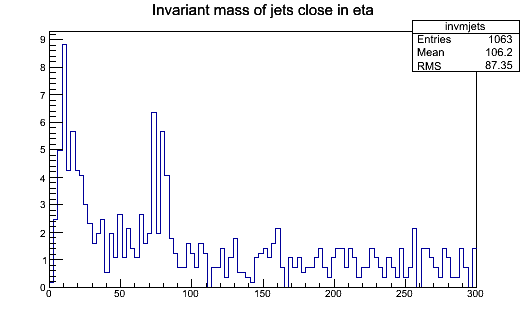# Analyzing Delta Eta for Jet Pairs

I am analyzing multi-jet events and need to find the jet pairs in each event which are close together in eta. The aim is to take an event and find a jet pair such that the eta difference between them goes from ±1 to ±2 and such that they are in a particular mass window, and then match the pair with a second pair which has the same delta Eta and a different mass window.

I can do the mass window part just by imposing a mass requirement and I am aware that I can obtain the eta value for a particular jet by using something like jet1->Eta, but I was unsure how to search for a pair with a particular eta difference, is there a Delta type function in C++ for a difference in a quantity as I have tried searching and did not find it.

Could you be a bit more specific? From the description above it’s unclear what this delta should be. For example, do you need to take phi into consideration? If you need a delta R function, here is an example in Python that you can easily convert to C++ (note, `hypot` below is the same as `std::hypot` in C++:

``````def deltaR(a,b):
dphi = abs(a.phi()-b.phi())
if dphi > pi: dphi = 2*pi-dphi
return hypot(a.eta()-b.eta(),dphi)
``````

What I’ve done is to write a piece of code to get a jet pair where the invariant mass and magnitude of eta are within a certain range:

``````if(njets&gt;3){

for(int i =0; i&lt;njets; i++){

j1 = (Jet *) branchJet-&gt;At(i);

if((j1-&gt;PT)&gt;30) {

for(int j = i; j &lt; njets; j++){

j2 = (Jet *) branchJet-&gt;At(j);

if((j2-&gt;PT)&gt;30  &amp;&amp; 70&lt;((jet1-&gt;P4()+jet2-&gt;P4()).M())&lt;100 &amp; 0&lt;abs(jet1-&gt;Eta - jet2-&gt;Eta)&lt;1)

invmjets-&gt;Fill((j1-&gt;P4()+j2-&gt;P4()).M(),norm);

}

}

}

}
``````

but it seems like it doesn’t do any calculations as nothing goes into the histogram and I can’t print off values for the invariant mass of the jets, is there something particular which is wrong with this code? I am unable to see what is really wrong with it.

Maybe you need to check your assumptions about the data. What happens if you relax the criteria a bit by increasing the ranges for eta and invariant mass, for example? Try to start with a loose cut and refine it as you go. What may be happening is that no event in your data is passing the requirements you are setting. In particular, you have `if (njets > 3)`, so if your events have only 2 or 3 jets each, which is not improbable, then you are rejecting all events just with this `if`.

I re-wrote and got results in a histogram but the results are a bit strange. The code I use is:

``````if (njets>3) {
for (int i =0; i<njets; i++) {
j1 = (Jet *) branchJet->At(i);
if ((j1->PT)>30) {
for (int j = 0; j < njets; j++) {
j2 = (Jet *) branchJet->At(j);
if ((j2->PT)>30  &&
((jet1->P4()+jet2->P4()).M())>70 &&
((jet1->P4()+jet2->P4()).M())<100 &&
abs((jet1->Eta) -(jet2->Eta))>0  &&
abs((jet1->Eta) - (jet2->Eta))<1) invmjets->Fill((j1->P4()+j2->P4()).M(),norm);
}
}
}
}
}
``````

However, the histogram has ranges of invariant mass below 70 even though that is the lower mass range, surely that doesn’t make sense?Sorry, I don’t know how I can help you further with debugging your code. Please try to discuss with someone that has familiarity with the data you are trying to process and your analysis (e.g., your professor/supervisor). The problem could be the data (e.g., trigger issue), a bug in how you are calculating some of the physical quantities, which jets you are selecting or otherwise filtering the data, etc, etc. The information above is not sufficient to identify the problem.

Note that, in the most internal loop, you use “jet1” and “jet2” but I think there should be “j1” and “j2” in all these places.
Also, in the most internal loop, I think you should use (otherwise you “double” pairs): `for (int j = (i + 1); j < njets; j++) {` (and then also `for (int i = 0; i < (njets - 1); i++) {` in the external loop) but you should also modify the first condition: `if ( ((j1->PT)>30 || (j2->PT)>30) &&`

This topic was automatically closed 14 days after the last reply. New replies are no longer allowed.